Published

# Coffee Alarm Clock

An alarm clock that only turns off when it senses that the lights have been turned on, and automatically starts brewing coffee.

BeginnerFull instructions provided5 hours987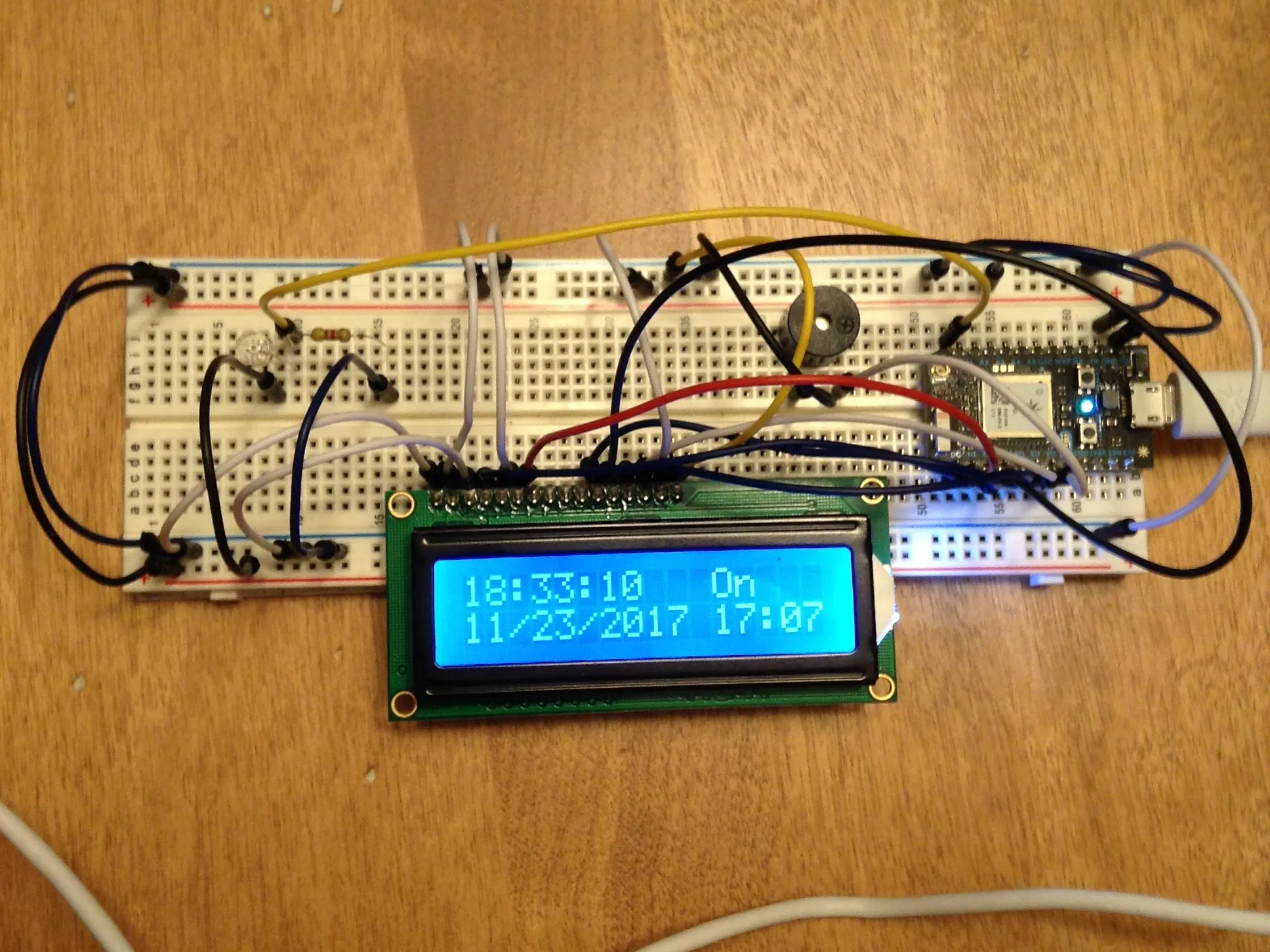## Things used in this project

### Hardware componentsParticle Photon
×2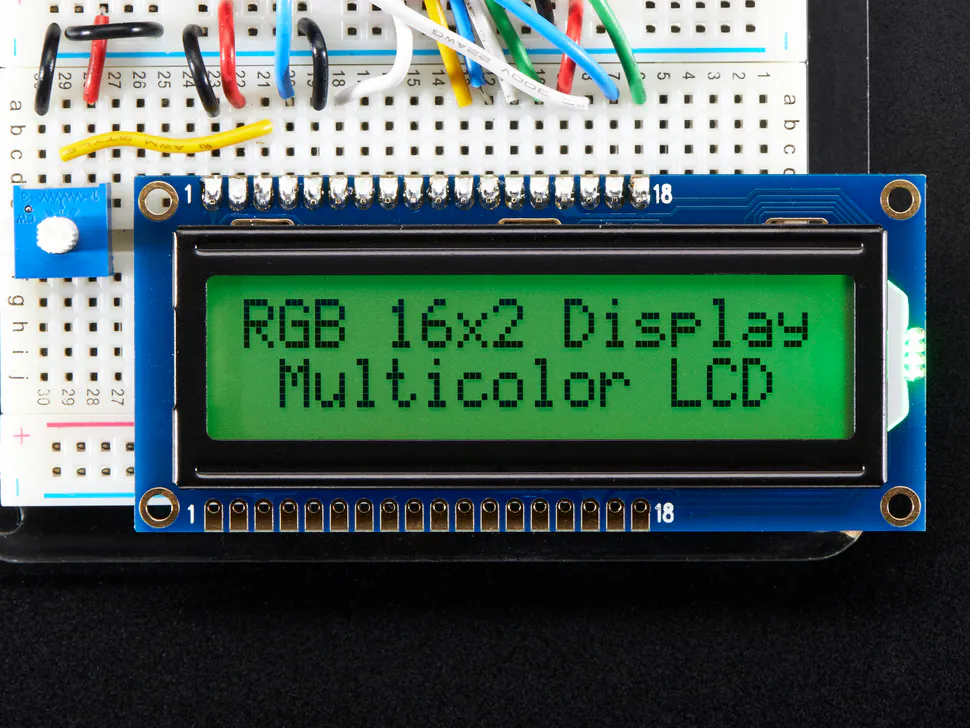Adafruit RGB Backlight LCD - 16x2
×1
 Servos (Tower Pro MG996R)
×1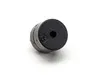Buzzer
×1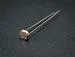Photo resistor
×1Jumper wires (generic)
×30Resistor 10k ohm
×1

### Software apps and online servicesIFTTT Maker serviceGoogle Sheets

Read more

## Schematics

### Schematic of the first Particle Photon.

See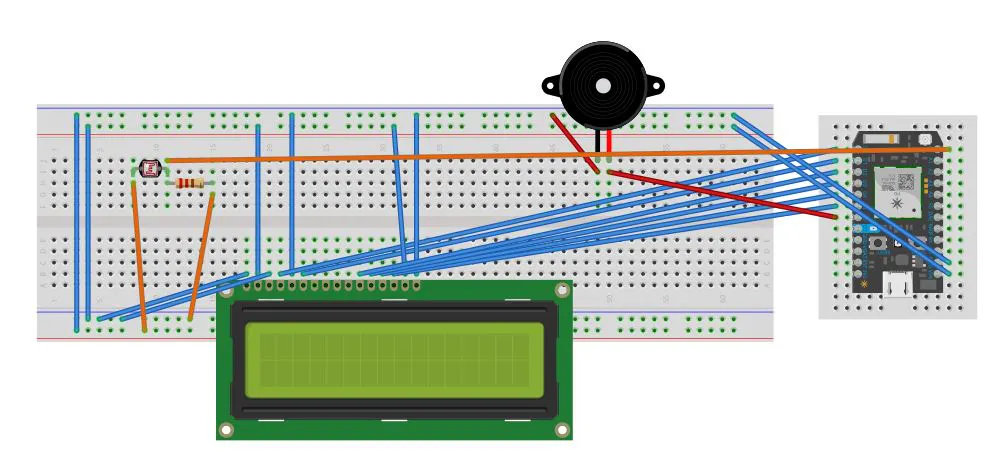### Schematic of the second particle photon

See below...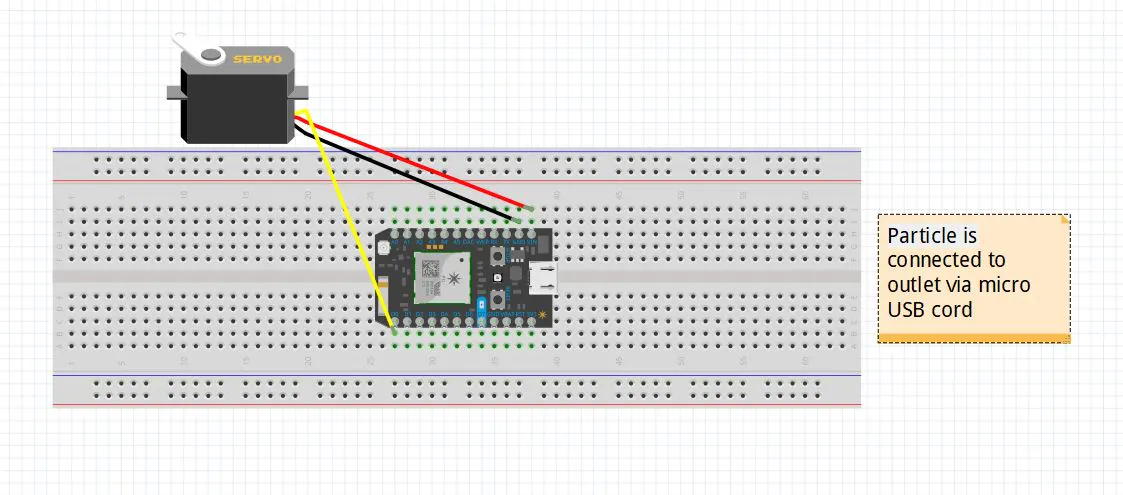## Code

### Second Particle Photon Code

C/C++
This code controls the Servo Motor.
```int servoPin=A0;
Servo myServo;
int servoPos=0;
int servoControl;

void setup() {
myServo.attach(D0);
Particle.function("servo", servoControl);
Particle.variable("servoPos", &servoPos, INT);

Serial.begin(3600);

}

void loop() {

}
{
int servoControl(String command)
int newPos=command.toInt();
servoPos=constrain(newPos,0,180);

myServo.write(servoPos);
return 1;

}
```

### LCD Particle

C/C++
This code controls the LCD, buzzer, and photocell.
```// This #include statement was automatically added by the Particle IDE.
#include <SparkTime.h>

// This #include statement was automatically added by the Particle IDE.
#include <LiquidCrystal.h>

// This #include statement was automatically added by the Particle IDE.
#include <LiquidCrystal_I2C_Spark.h>

// This #include statement was automatically added by the Particle IDE.
#include <Adafruit_DHT.h>

#include <application.h>

#define DHTPIN 5
#define DHTTYPE DHT11

int pos = 0;
int light;
int light_sensor_pin = A0;

LiquidCrystal lcd(D0, D1, D2, D3, D4, D5);
SparkTime rtc;
UDP UDPClient;
unsigned long currentTime;
int alarmhour = 0;
int alarmmin = 0;
int alarmsec = 0;
bool alarm = false;

DHT dht(DHTPIN, DHTTYPE);

void setup()
{
dht.begin();
Particle.function("Timer", tim);
Particle.function("Set", set);
Particle.function("Preset", clk);
rtc.begin(&UDPClient, "time.nist.gov");
rtc.setTimeZone(-5); // gmt offset
pinMode(D6, OUTPUT);
lcd.begin(16,2);
}

////////////////////////////////Command for custom timer times////////////////////////
int tim(String pTim)
//int gong(String command)
{
const char* str = pTim;
int myNr;

if(sscanf(str, "%d", &myNr)  == EOF )
{
/* error */
}
alarm = true;
currentTime = rtc.now();
int min = rtc.minute(currentTime);  //sets time
int hour = rtc.hour(currentTime);
int sec = rtc.second(currentTime);
alarmhour = (hour + myNr/100);
alarmmin = (min + myNr % 100);
if(alarmmin > 60)
{
alarmhour+=1;
alarmmin-=60;
}
if(alarmhour >= 24)
{
alarmhour = 0;
}
}
//////////////////////////////Command for custom alarm times///////////////////////////
int set(String pTime)   // when "gong" is called from the cloud, it will
{
const char* str = pTime;
int myNr;

if(sscanf(str, "%d", &myNr)  == EOF )
{
/* error */
}
int pHour = myNr / 100;
int pMin = myNr % 100;
alarm = true;
alarmhour = pHour;
alarmmin = pMin;
}
/////////////////////////////////Command for pre set alarm times//////////////////////////
int clk(String command)
{

if(command == "School")
{
alarm = true;
alarmhour = 6;
alarmmin = 0;
}

if(command == "Weekend")
{
alarm = true;
alarmhour = 11;
alarmmin = 38;
}
/*if(command == "Off")
{
alarm = false;
alarmhour = 0;
alarmmin = 0;
lcd.clear();
}*/

}

void loop()
{

currentTime = rtc.now();
int min = rtc.minute(currentTime);  //sets time
int hour = rtc.hour(currentTime);
int sec = rtc.second(currentTime);

int day = rtc.day(currentTime);
int month = rtc.month(currentTime);
int year = rtc.year(currentTime);
///////////////////////////////Print Time////////////////////////////////////

if(hour < 10) {
lcd.setCursor(0,0);
lcd.print("0");
lcd.setCursor(1,0);
lcd.print(hour);
}
if(hour >= 10) {
lcd.setCursor(0,0);
lcd.print(hour);
}

lcd.setCursor(2,0);
lcd.print(":");

if(min < 10) {
lcd.setCursor(3,0);
lcd.print("0");
lcd.setCursor(4,0);
lcd.print(min);
}
if(min >= 10) {
lcd.setCursor(3,0);
lcd.print(min);
}

lcd.setCursor(5,0);
lcd.print(":");

if(sec < 10) {
lcd.setCursor(6,0);
lcd.print("0");
lcd.setCursor(7,0);
lcd.print(sec);
}
if(sec >= 10) {
lcd.setCursor(6,0);
lcd.print(sec);
}

lcd.setCursor(0,1);
lcd.print(month);

lcd.print("/");
lcd.print(day);

lcd.print("/");
lcd.print(year);

////////////////////////////Alarm print/////////////////////////////

if(alarm == false)
{
lcd.setCursor(11,0);
lcd.print("A:No ");
}
else
{
lcd.setCursor(11,0);
lcd.print("A:Set");

if(alarmhour < 10)
{
lcd.setCursor(11,1);
lcd.print("0");
lcd.setCursor(12,1);
lcd.print(alarmhour);
}
if(alarmhour >= 10)
{
lcd.setCursor(11,1);
lcd.print(alarmhour);
}

lcd.setCursor(13,1);
lcd.print(":");

if(alarmmin < 10)
{
lcd.setCursor(14,1);
lcd.print("0");
lcd.setCursor(15,1);
lcd.print(alarmmin);
}
if(alarmmin >= 10)
{
lcd.setCursor(14,1);
lcd.print(alarmmin);
}
}

if(alarmhour == hour)
{
if(alarmmin == min)
{
while(sec<5)
{
buzzer();
sec+=50;
}
}
}
float light_measurement = analogRead(light_sensor_pin);
light = (int)(light_measurement/4096*100);

// Publish data
Spark.publish("light", String(light) + "%");
delay(1000);
}

void buzzer()
{
if(light>10)
{digitalWrite(D6, LOW);
}
else{
digitalWrite(D6,LOW);
}
for(int i = 0; i < 5; i++)
{
delay(50);
digitalWrite(D6, HIGH);
delay(450);
digitalWrite(D6, LOW);
delay(500);
}
}
```

## Credits

### Caleb Eng

1 project • 1 follower